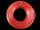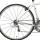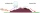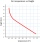# Direct relationship + fundamentals of Physics - examples

1. Electrics - conductorThe wire is 106 meters long at 0 °C and at every temperature increase of 1 °C the length increases by 0.15 mm per 1 m length of wire. Determine a function which represents the overall length of the wire as a function of temperature. What is the length of t
2. Tower modelTower height is 300 meters, weight 8000 tons. How high is the model of the tower weight 1 kg? (State the result in the centimeters). The model is made from exactly the same material as the original no numbers need to be rounded. The result is a three-digi
3. Bicycle gearsThe toothed wheel on the bicycle pedal has 40 teeth, the wheel on the rear wheel has only 16 teeth. How many times does the rear wheel turn if the pedals rotate 50 times?
4. SeawaterSeawater has a density of 1025 kg/m3, ice 920 kg/m3. 8 liters of seawater froze and created a cube. Calculate the size of the cube edge.
5. ImagineImagine that a unit of air at a temperature of 25°C rises up a mountain range that is 3,000 meters high on the windward side and which descends to 1,200 meters on the leeward side, assuming that the air will remain dry what will its temperature when it cro
6. Air thermalImagine that a unit of air rises at 3000 meters high, if temperature decreases 6 degrees celcius for every 1000 meter, what will be its temperature at 1400 meters, 2000 meters, 2500 meters and when it reaches the 3000 meter elevation. Starting temperature.

We apologize, but in this category are not a lot of examples.
Do you have an interesting mathematical example that you can't solve it? Enter it, and we can try to solve it.

To this e-mail address, we will reply solution; solved examples are also published here. Please enter e-mail correctly and check whether you don't have a full mailbox.